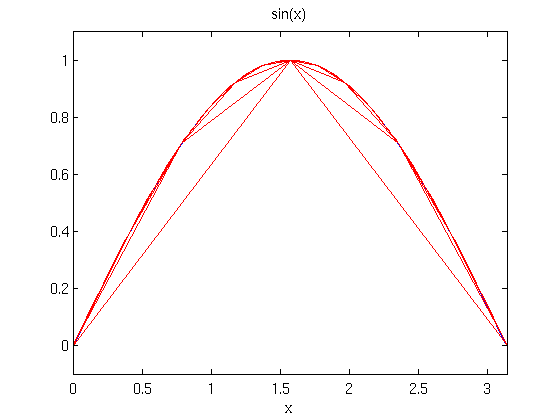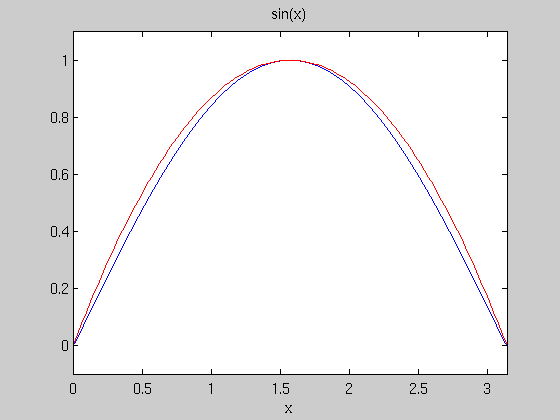# Demo on the Trapezoidal Rule and Simpson's Rule

## The Trapezoidal Rule

The idea of the trapezoidal rule is to approximate a general curve by trapezoids, like this. We illustrate with the problem of integrating sin(x) from 0 to pi. Of course, the true value of the integral is 2.

```ezplot('sin(x)', [0, pi]), hold on
approx = zeros(1,7); %initialize vector of results
for j = 1:7
n = 2^j;
x = pi*(0:1/n:1);
plot(x, sin(x), 'r')
weights = [1, 2*ones(1,n-1), 1];
approx(j) = pi/(2*n)*sin(x)*weights';
end
disp('Using Trapezoidal Rule')
disp('         n       Approximation')
for j = 1:7
disp(['n = ', num2str(2^j, '%d'), '    ', num2str(approx(j), '%1.10f')])
end
```
```Using Trapezoidal Rule
n       Approximation
n = 2    1.5707963268
n = 4    1.8961188979
n = 8    1.9742316019
n = 16    1.9935703438
n = 32    1.9983933610
n = 64    1.9995983886
n = 128    1.9998996002
```## Simpson's Rule

The idea of Simpson's rule is to approximate a general curve by arcs of parabolas, like this. We illustrate with the problem of integrating sin(x) from 0 to pi. Of course, the true value of the integral is 2.

```figure, ezplot('sin(x)', [0, pi]), hold on
x = 0:.01:1;
plot(pi*x, 1 - 4*(x-.5).^2, 'r')
approx = zeros(1, 7); %initialize vector of results
for j = 1:7
n = 2^j;
x = pi*(0:1/n:1);
weights = [1,2*ones(1,n-1)+2*mod([1:n-1],2),1] ;
approx(j) = pi/(3*n)*sin(x)*weights';
end
disp('Using Simpson Rule')
disp('         n       Approximation')
for j = 1:7
disp(['n = ', num2str(2^j, '%d'), '    ', num2str(approx(j), '%1.10f')])
end
```
```Using Simpson Rule
n       Approximation
n = 2    2.0943951024
n = 4    2.0045597550
n = 8    2.0002691699
n = 16    2.0000165910
n = 32    2.0000010334
n = 64    2.0000000645
n = 128    2.0000000040
```Note that for smooth functions, the error in Simpson's rule is roughly proportional to 1/n^4, so that it doesn't require a large value of n to get reasonable accuracy. However, for very jagged functions, the trapezoidal rule can be more accurate. Here is a program to compute the Simpson's rule approximation to an integral, along with some examples.

```type Simpson
```
```function Q = Simpson(fun, a, b, n)
%SIMPSON Numerically evaluate integral,  using Simpson's rule.
% syntax: Q = Simpson(fun, a, b, n)
% FUN should be a vectorized function defined on the interval
% from a to b.  n should be a positive even integer.
% example: Simpson(@sin, 0, pi,4) returns the approximation
% 2.0046 to the integral 2 of the sine function from 0 to pi.
if mod(n,2)
error('n must be even')
end;
weights = [1,2*ones(1,n-1)+2*mod([1:n-1],2),1] ;
x = a:(b-a)/n:b;
Q = (b-a)/(3*n)*fun(x)*weights';
return
```
```Simpson(@(x) x.^3, 0, 1, 2)
```
```ans =

0.2500

```

The above example shows that Simpson's rule is perfectly accurate for cubic polynomial functions. On the other hand, the square root function has a derivative that blows up at x= 0, so in this case the results won't be as good, even with a much bigger value of n:

```Simpson(@sqrt, 0, 1, 2)
```
```ans =

0.6381

```
```Simpson(@sqrt, 0, 1, 16)
```
```ans =

0.6654

```

The true value of the ingral of sqrt(x) from 0 to 1 is 2/3, so the error as a function of n looks like:

```for j = 1:10
disp(['n = ', num2str(2^j, '%d'), '    ', 'error =', ...
num2str(2/3 - Simpson(@sqrt, 0, 1, 2^j), '%1.10f')])
end
```
```n = 2    error =0.0285954792
n = 4    error =0.0101404019
n = 8    error =0.0035873866
n = 16    error =0.0012684780
n = 32    error =0.0004484839
n = 64    error =0.0001585636
n = 128    error =0.0000560607
n = 256    error =0.0000198205
n = 512    error =0.0000070076
n = 1024    error =0.0000024776
```

One can see from this example that doubling n still decreases the error by more than a factor of 2, but by less than a factor of 16, more like a factor of 3.